# RSS vs TSS vs R-square

## RSS vs TSS vs R-square

Residual Sum of Square (RSS) is the sum of squares of error terms and this is an absolute number. This number can vary greatly based on the number of data points. RSS will increase if the data points are more and the value will decrease If the data points are less.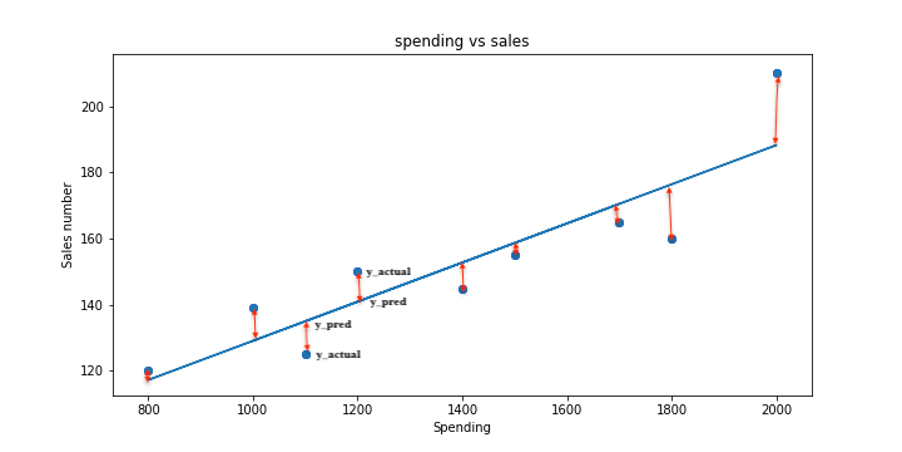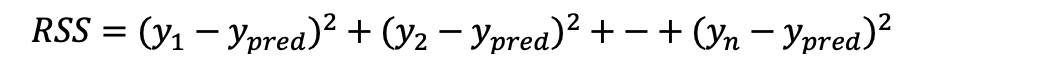### TSS

The total sum of squares (TSS) is a variation from the actual value from the mean value of data points.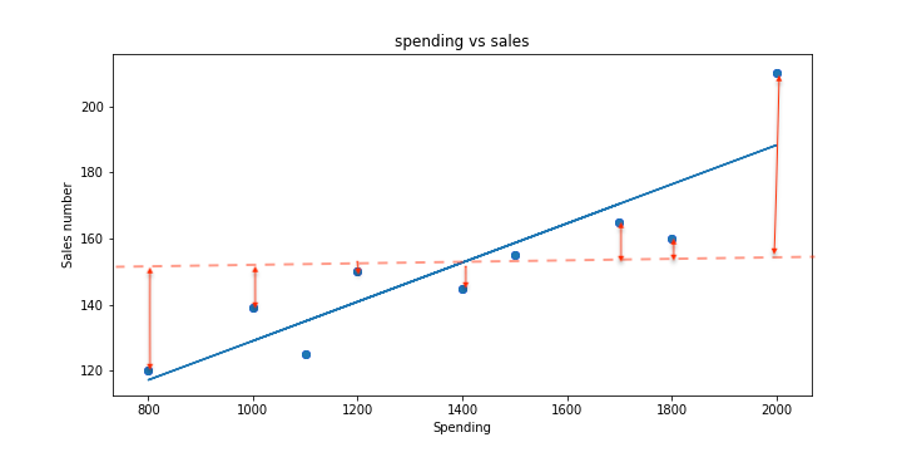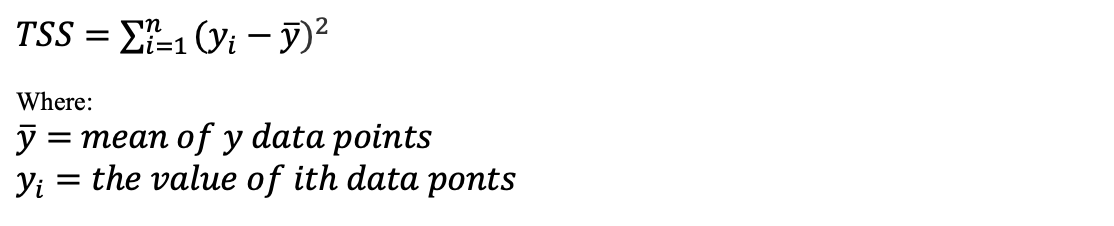### R-squared

R-sq is a measure of variance for dependent variables. That is variance in the output that is explained by the small change in input.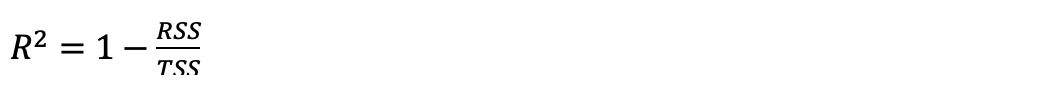The value of R-sq is always between 0 (0%) and 1 (100%). The bigger the value better the fit.